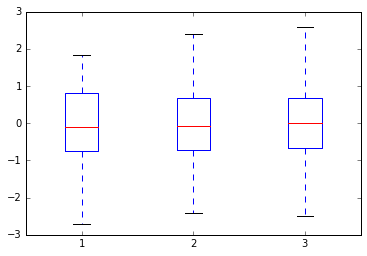In :
import numpy as np
import matplotlib.pyplot as plt

# notebook command to show the plot in the browser
%matplotlib inline

x = np.linspace(0,2 *np.pi, 100)

Sin = np.sin(x)
Cos = np.cos(x)

plt.plot(x, Sin)
plt.plot(x,Cos)

Out:
[<matplotlib.lines.Line2D at 0x10a478f28>]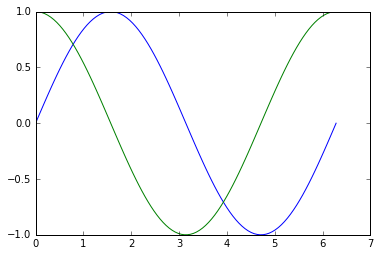In :
import numpy as np
import matplotlib.pyplot as plt

# notebook command to show the plot in the browser
%matplotlib inline

data = np.random.randn(1024,2)

plt.scatter(data[:,0], data[:,1])
plt.show()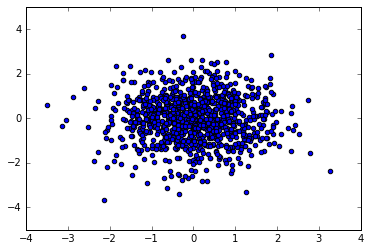In :
# Plotting multiple bars (grouped bars)

import numpy as np
import matplotlib.pyplot as plt

# notebook command to show the plot in the browser
%matplotlib inline

data = [ [5,15,20,50] , [8,9,20,40] , [3,16,30,10] ]
num_bars = len(data)
color_list = ['b', 'g', 'r']

gap = 0.2                         # space each bars group 0.2 from the next group
bar_width = (1- gap) / num_bars   # divide the remaining distance equally between the bars

for i, row in enumerate(data):    # enumerate returns a data unit (a row) and its index
x = np.arange(len(row))
plt.bar(x+i *bar_width, row,       # blue starts at x=0, i=0 => 0
#                          # green starts at x=0, i=1 => 0.2
#                          # red starts at   x=0, i=2 => 0.4
width=bar_width,
color = color_list[i % len(color_list)])

plt.show

Out:
<function matplotlib.pyplot.show>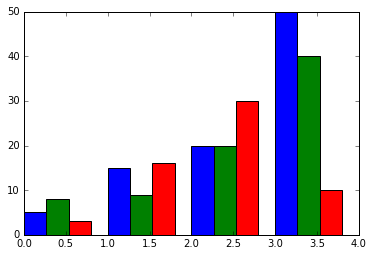In :
# Plotting stacked bars

import matplotlib.pyplot as plt

# notebook command to show the plot in the browser
%matplotlib inline

d1 = [1,3,5,7]
d2 = [4,5,7,8]

x = range(len(d1))

plt.bar(x, d1, color='b')           # the function bar is used to plot bar charts
plt.bar(x, d2, color='r', bottom=d1)# the optional parammeter bottom specifies the starting point for bars

plt.show()

Out:
<Container object of 4 artists>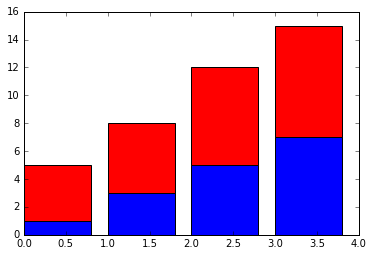In :
# Plotting stacked bars

import matplotlib.pyplot as plt
import numpy as np
# notebook command to show the plot in the browser
%matplotlib inline

"""
Why we need numpy in this example

numpy enables us to create arrays;
numpay.array1 + numpay.array2 = numpay.array3 (this is an element by elemnet addition)

Python creates lists;
list1 + list2 is a concatintion.
If we want to add two list we need to iterate overthem and add element by elemnet
"""

d1 = np.array([1,3,5,7])
d2 = np.array([4,5,7,8])
d3 = np.array([3,6,1,8])

x = range(len(d1))

plt.bar(x, d1, color='b')
plt.bar(x, d2, color='r', bottom=d1)
plt.bar(x, d3, color='g', bottom=(d1+d2))  # with help of numpy d1+d2 is the correct base for the third stack of bar

plt.show()

Out:
<Container object of 4 artists>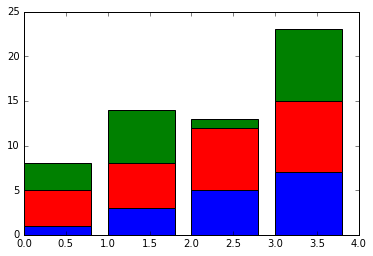In :
# Plotting horizontal bars (with back to back)

import matplotlib.pyplot as plt
import numpy as np     # needed to do array operations ( to eliminate using lists and iterate overthem)

# notebook command to show the plot in the browser
%matplotlib inline

d1 = np.array([1,3,5,7])
d2 = np.array([4,5,7,8])

x = range(len(d1))

plt.barh(x, d1, color='b')           # the function bar is used to plot bar charts
plt.barh(x, -d2, color='r')          # the optional parammeter bottom specifies the starting point for bars
plt.show()

Out:
<Container object of 4 artists>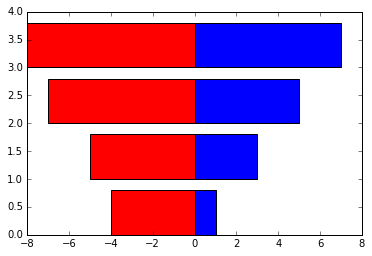In :
# Plotting pie chart
"""
Pie chart is used to show the relative importance/size of quantities that are grouped together.
"""
import matplotlib.pyplot as plt

# notebook command to show the plot in the browser
%matplotlib inline

d1 = [1,3,5,7]

plt.pie(d1)
plt.show()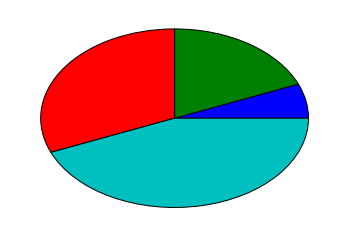In :
#plotting histograms
"""
histogram is used to plot the frequency of occurrence (how many something is repeated)
"""
import matplotlib.pyplot as plt
import numpy as np

# notebook command to show the plot in the browser
%matplotlib inline

# generate random normally distributed data with mean =0  and variance = 1
data = np.random.randn(10000)

plt.hist(data, bins=20) # plot a histogram with 20 bins
plt.show()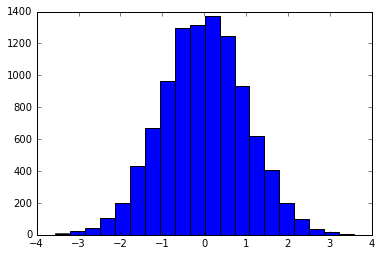In :
import numpy as np
import matplotlib.pyplot as plt

# notebook command to show the plot in the browser
%matplotlib inline

data = np.random.randn(100,3)

plt.boxplot(data)
plt.show()